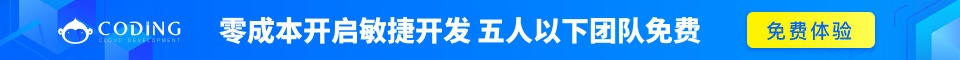# Ruby 方法

Ruby 方法与其他编程语言中的函数类似。Ruby 方法用于捆绑一个或多个重复的语句到一个单元中。

## 语法

```def method_name [( [arg [= default]]...[, * arg [, &expr ]])]
expr..
end
```

```def method_name
expr..
end
```

```def method_name (var1, var2)
expr..
end
```

```def method_name (var1=value1, var2=value2)
expr..
end
```

```method_name
```

```method_name 25, 30
```

## 实例

```#!/usr/bin/ruby

def test(a1="Ruby", a2="Perl")
puts "The programming language is #{a1}"
puts "The programming language is #{a2}"
end
test "C", "C++"
test
```

```The programming language is C
The programming language is C++
The programming language is Ruby
The programming language is Perl
```

## 从方法返回值

Ruby 中的每个方法默认都会返回一个值。这个返回的值是最后一个语句的值。例如：

```def test
i = 100
j = 10
k = 0
end
```

## Ruby return 语句

Ruby 中的 return 语句用于从 Ruby 方法中返回一个或多个值。

## 语法

```return [expr[`,' expr...]]
```

## 实例

```return

OR

return 12

OR

return 1,2,3
```

```#!/usr/bin/ruby

def test
i = 100
j = 200
k = 300
return i, j, k
end
var = test
puts var
```

```100
200
300
```

## 可变数量的参数

```#!/usr/bin/ruby

def sample (*test)
puts "The number of parameters is #{test.length}"
for i in 0...test.length
puts "The parameters are #{test[i]}"
end
end
sample "Zara", "6", "F"
sample "Mac", "36", "M", "MCA"
```

```The number of parameters is 3
The parameters are Zara
The parameters are 6
The parameters are F
The number of parameters is 4
The parameters are Mac
The parameters are 36
The parameters are M
The parameters are MCA
```

## 类方法

Ruby 提供了一种不用实例化类即可访问方法的方式。让我们看看如何声明并访问类方法：

```class Accounts
end
def Accounts.return_date
end
end
```

```Accounts.return_date
```

## 语法

```alias method-name method-name
alias global-variable-name global-variable-name
```

## 实例

```alias foo bar
alias \$MATCH \$&
```

## 语法

```undef method-name
```

## 实例

```undef bar
```